# 1.4 Inverse functions  (Page 3/11)

 Page 3 / 11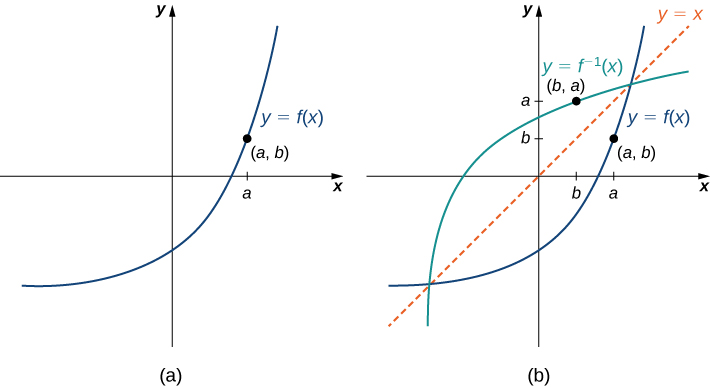(a) The graph of this function f shows point ( a , b ) on the graph of f . (b) Since ( a , b ) is on the graph of f , the point ( b , a ) is on the graph of f −1 . The graph of f −1 is a reflection of the graph of f about the line y = x .

## Sketching graphs of inverse functions

For the graph of $f$ in the following image, sketch a graph of ${f}^{-1}$ by sketching the line $y=x$ and using symmetry. Identify the domain and range of ${f}^{-1}.$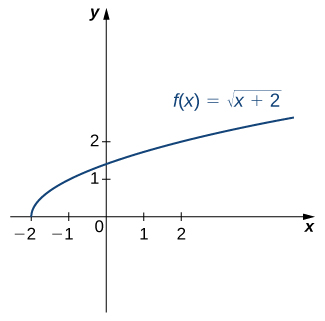Reflect the graph about the line $y=x.$ The domain of ${f}^{-1}$ is $\left[0,\infty \right).$ The range of ${f}^{-1}$ is $\left[-2,\infty \right).$ By using the preceding strategy for finding inverse functions, we can verify that the inverse function is ${f}^{-1}\left(x\right)={x}^{2}-2,$ as shown in the graph.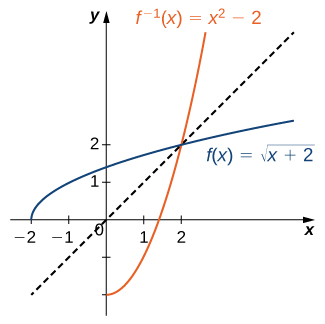Sketch the graph of $f\left(x\right)=2x+3$ and the graph of its inverse using the symmetry property of inverse functions.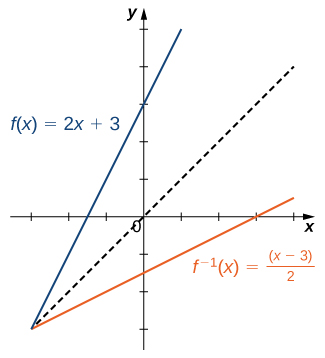## Restricting domains

As we have seen, $f\left(x\right)={x}^{2}$ does not have an inverse function because it is not one-to-one. However, we can choose a subset of the domain of $f$ such that the function is one-to-one. This subset is called a restricted domain    . By restricting the domain of $f,$ we can define a new function $g$ such that the domain of $g$ is the restricted domain of $f$ and $g\left(x\right)=f\left(x\right)$ for all $x$ in the domain of $g.$ Then we can define an inverse function for $g$ on that domain. For example, since $f\left(x\right)={x}^{2}$ is one-to-one on the interval $\left[0,\infty \right),$ we can define a new function $g$ such that the domain of $g$ is $\left[0,\infty \right)$ and $g\left(x\right)={x}^{2}$ for all $x$ in its domain. Since $g$ is a one-to-one function, it has an inverse function, given by the formula ${g}^{-1}\left(x\right)=\sqrt{x}.$ On the other hand, the function $f\left(x\right)={x}^{2}$ is also one-to-one on the domain $\left(\text{−}\infty ,0\right].$ Therefore, we could also define a new function $h$ such that the domain of $h$ is $\left(\text{−}\infty ,0\right]$ and $h\left(x\right)={x}^{2}$ for all $x$ in the domain of $h.$ Then $h$ is a one-to-one function and must also have an inverse. Its inverse is given by the formula ${h}^{-1}\left(x\right)=\text{−}\sqrt{x}$ ( [link] ).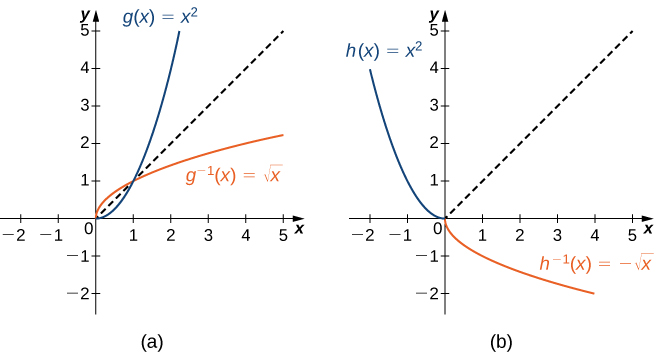(a) For g ( x ) = x 2 restricted to [ 0 , ∞ ) , g −1 ( x ) = x . (b) For h ( x ) = x 2 restricted to ( − ∞ , 0 ] , h −1 ( x ) = − x .

## Restricting the domain

Consider the function $f\left(x\right)={\left(x+1\right)}^{2}.$

1. Sketch the graph of $f$ and use the horizontal line test to show that $f$ is not one-to-one.
2. Show that $f$ is one-to-one on the restricted domain $\left[-1,\infty \right).$ Determine the domain and range for the inverse of $f$ on this restricted domain and find a formula for ${f}^{-1}.$
1. The graph of $f$ is the graph of $y={x}^{2}$ shifted left 1 unit. Since there exists a horizontal line intersecting the graph more than once, $f$ is not one-to-one.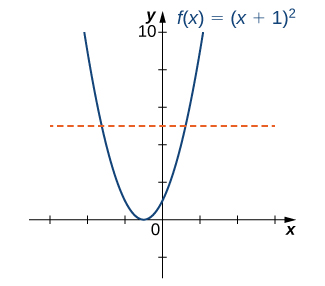2. On the interval $\left[-1,\infty \right),\phantom{\rule{0.2em}{0ex}}f$ is one-to-one.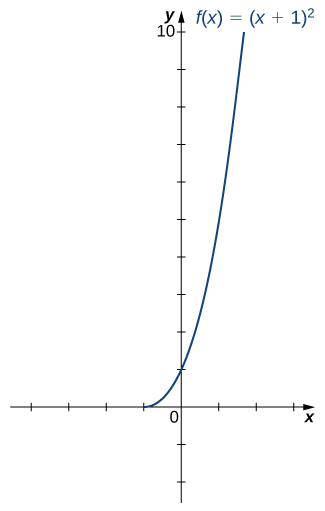The domain and range of ${f}^{-1}$ are given by the range and domain of $f,$ respectively. Therefore, the domain of ${f}^{-1}$ is $\left[0,\infty \right)$ and the range of ${f}^{-1}$ is $\left[-1,\infty \right).$ To find a formula for ${f}^{-1},$ solve the equation $y={\left(x+1\right)}^{2}$ for $x.$ If $y={\left(x+1\right)}^{2},$ then $x=-1±\sqrt{y}.$ Since we are restricting the domain to the interval where $x\ge -1,$ we need $±\sqrt{y}\ge 0.$ Therefore, $x=-1+\sqrt{y}.$ Interchanging $x$ and $y,$ we write $y=-1+\sqrt{x}$ and conclude that ${f}^{-1}\left(x\right)=-1+\sqrt{x}.$

Consider $f\left(x\right)=1\text{/}{x}^{2}$ restricted to the domain $\left(\text{−}\infty ,0\right).$ Verify that $f$ is one-to-one on this domain. Determine the domain and range of the inverse of $f$ and find a formula for ${f}^{-1}.$

The domain of ${f}^{-1}$ is $\left(0,\infty \right).$ The range of ${f}^{-1}$ is $\left(\text{−}\infty ,0\right).$ The inverse function is given by the formula ${f}^{-1}\left(x\right)=-1\text{/}\sqrt{x}.$

## Inverse trigonometric functions

The six basic trigonometric functions are periodic, and therefore they are not one-to-one. However, if we restrict the domain of a trigonometric function to an interval where it is one-to-one, we can define its inverse. Consider the sine function ( [link] ). The sine function is one-to-one on an infinite number of intervals, but the standard convention is to restrict the domain to the interval $\left[-\frac{\pi }{2},\frac{\pi }{2}\right].$ By doing so, we define the inverse sine function on the domain $\left[-1,1\right]$ such that for any $x$ in the interval $\left[-1,1\right],$ the inverse sine function tells us which angle $\theta$ in the interval $\left[-\frac{\pi }{2},\frac{\pi }{2}\right]$ satisfies $\text{sin}\phantom{\rule{0.1em}{0ex}}\theta =x.$ Similarly, we can restrict the domains of the other trigonometric functions to define inverse trigonometric functions    , which are functions that tell us which angle in a certain interval has a specified trigonometric value.

f(x) = x-2 g(x) = 3x + 5 fog(x)? f(x)/g(x)
fog(x)= f(g(x)) = x-2 = 3x+5-2 = 3x+3 f(x)/g(x)= x-2/3x+5
diron
pweding paturo nsa calculus?
jimmy
how to use fundamental theorem to solve exponential
find the bounded area of the parabola y^2=4x and y=16x
what is absolute value means?
Chicken nuggets
Hugh
🐔
MM
🐔🦃 nuggets
MM
(mathematics) For a complex number a+bi, the principal square root of the sum of the squares of its real and imaginary parts, √a2+b2 . Denoted by | |. The absolute value |x| of a real number x is √x2 , which is equal to x if x is non-negative, and −x if x is negative.
Ismael
find integration of loge x
find the volume of a solid about the y-axis, x=0, x=1, y=0, y=7+x^3
how does this work
Can calculus give the answers as same as other methods give in basic classes while solving the numericals?
log tan (x/4+x/2)
Rohan
Rohan
y=(x^2 + 3x).(eipix)
Claudia
Ismael
A Function F(X)=Sinx+cosx is odd or even?
neither
David
Neither
Lovuyiso
f(x)=1/1+x^2 |=[-3,1]
apa itu?
fauzi
determine the area of the region enclosed by x²+y=1,2x-y+4=0
Hi
MP
Hi too
Vic
hello please anyone with calculus PDF should share
Which kind of pdf do you want bro?
Aftab
hi
Abdul
can I get calculus in pdf
Abdul
explain for me
Usman
okay I have such documents
Fitzgerald
Hamza
How to use it to slove fraction
Hello please can someone tell me the meaning of this group all about, yes I know is calculus group but yet nothing is showing up
Shodipo
You have downloaded the aplication Calculus Volume 1, tackling about lessons for (mostly) college freshmen, Calculus 1: Differential, and this group I think aims to let concerns and questions from students who want to clarify something about the subject. Well, this is what I guess so.
Jean
Im not in college but this will still help
nothing
how en where can u apply it
Migos
how can we scatch a parabola graph
Ok
Endalkachew
how can I solve differentiation?
with the help of different formulas and Rules. we use formulas according to given condition or according to questions
CALCULUS
For example any questions...
CALCULUS
v=(x,y) وu=(x,y ) ∂u/∂x* ∂x/∂u +∂v/∂x*∂x/∂v=1
log tan (x/4+x/2)
Rohan
what is the procedures in solving number 1?

#### Get Jobilize Job Search Mobile App in your pocket Now!By Edgar DelgadoBy Caitlyn GobbleBy Nick SwainBy Zarina ChocolateBy Madison ChristianBy Angela EckmanBy OpenStaxBy OpenStaxBy Brooke DelaneyBy Dewey Compton